Next: Bibliography Up: Optimal Scoring Matrices for Previous: Degrees of Freedom

# Example

We use a random matrix M as an example. For simplicity we will assume that we have only 4 amino acids, i.e. M will be a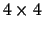matrix. M is generated to satisfy the normal properties: the columns should add to 1 and the pseudo-symmetry condition fi Mji = fj Mij must hold.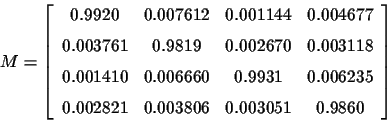The frequency vector f can be computed from the eigenvector of M whose eigenvalue is 1 or from the first row/column of M .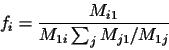The eigenvalues of M are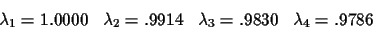In this case we want to find the optimal scoring matrix for d in the range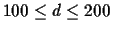. After setting E11=-1 and E12=1 and numerically minimizing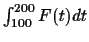on the rest of the Eij unknowns, we obtain: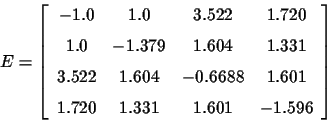From these values, using equation 3, we can compute

S(d) = 1.258-.3307(.9786)d-1.459(.9914)d-.5098(.9830)d

The derivative of S(d) with respect to d is:

S'(d) = .007136(.9786)d+.01264(.9914)d+.008734(.9830)d

and we can see that all the terms are strictly positive, so S(d) is strictly monotonic for all d. Suppose we have aligned these two sequences:The score for this alignment is

w/n = (E33+E24+E11+E23+E41+E31+E42+E22)/8 =.8076

From this value, and using any numerical method, we can find the d* which satisfies the equation

S(d*) = .8076

The solution is

d*=149.99

The variance of the estimator is. The variance is very large, and so is the standard deviation (127.34), which is not unexpected for such a short alignment.Next: Bibliography Up: Optimal Scoring Matrices for Previous: Degrees of Freedom
Chantal Korostensky
1999-07-14